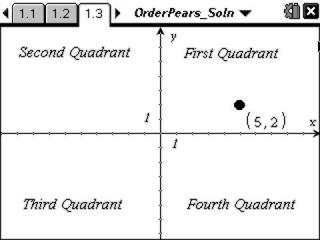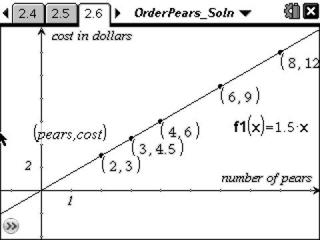# Activities

••• ##### Subject Area

• Math: Algebra I: Quadratic Functions

• ##### Author9-12

15 Minutes

• ##### Device
• TI-Nspire™ Navigator™
• TI-Nspire™
• TI-Nspire™ CAS
• ##### Software

TI-Nspire™
TI-Nspire™ CAS

2.0

## Order Pears#### Activity Overview

In this activity, students will interactively investigate ordered pairs. They will graphically explore the coordinates of a point on a Cartesian plane, identifying characteristics of a point corresponding to the coordinate. Students will plot ordered pairs of a function, list these in a table of values, and graph them in a scatter plot.

#### Key Steps

•In this short activity, students explore an ordered pair on the coordinate plane. Then they will answer self-check questions about the quadrant of a specific point and general combinations of positive and negative ordered pairs. Students will plot points with letters on the coordinate plane and when correctly plotted will reveal a phrase.

•Students will then given a function for the cost of ordering pears. They will enter ordered pairs into and spreadsheet and observe the pattern as the ordered pairs appear in a scatter plot. Then students will add the line y=x and rotate it so the line passes through the ordered pairs, noting the slope.